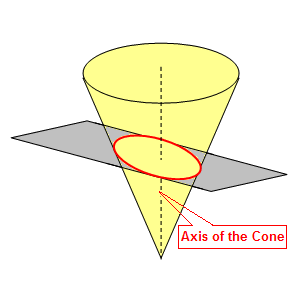# Ellipse

An ellipse is the set of all points $P$ in a plane such that the sum of the distances from $P$ to two fixed points is a given constant.  Each of the fixed points is called a focus .  (The plural is foci.)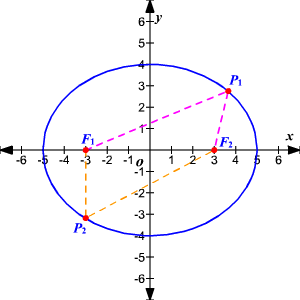The segments $\stackrel{¯}{P{F}_{1}}$  and $\stackrel{¯}{P{F}_{2}}$ are the focal radii of $P$ .

The center of the ellipse is the midpoint of the line segment joining its foci. The major axis of the ellipse is the chord that passes through its foci and has its endpoints on the ellipse. The minor axis of the ellipse is the chord that contains the center of the ellipse, has its endpoints on the ellipse and is perpendicular to the major axis.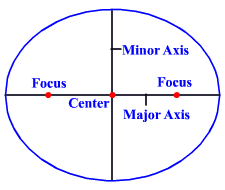An ellipse has a quadratic equation in two variables.

Given an ellipse with its center at $\left(0,0\right)$ , its foci on the $x$ -axis at $\left(c,0\right)$ and $\left(-c,0\right)$ , $x$ -intercepts $\left(±a,0\right)$ and $x$ -intercepts $\left(0,±b\right)$ .  The length of the major axis is $2a$ and the equation of the ellipse is

The major axis is on the $x$ -axis.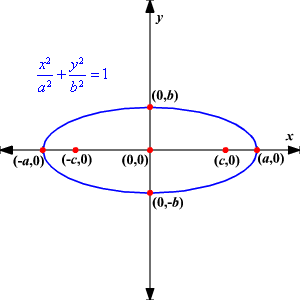If the foci on the ellipse are on the $y$ -axis, then the focal points are $\left(0,±c\right)$ , and the formula is

The major axis is on the $y$ -axis.  The $x$ -intercepts are $\left(±b,0\right)$ and the $y$ -intercepts are $\left(0,±a\right)$ .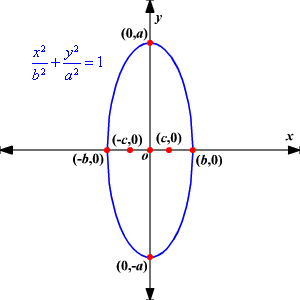Notice that the major axis is horizontal if the ${x}^{2}$ -term has the larger denominator and vertical if the ${y}^{2}$ -term has the larger denominator.  Since the larger of the two denominators is ${a}^{2}$ , the length of the major axis is always $2a$ and the length of the minor axis is always $2b$ .  The distance from the center to either focus is | $c$ |.

Since the center of each of these ellipses has its center on the origin, they are called central ellipses .

Example 1:

Given the ellipse with the equation $\frac{{x}^{2}}{9}+\frac{{y}^{2}}{4}=1$ , find its
$x$ - and $y$ -intercepts and its foci.

Its $x$ -intercepts are $\left(3,0\right)$ and $\left(-3,0\right)$ .

Its $y$ -intercepts are $\left(0,2\right)$ and $\left(0,-2\right)$ .

${b}^{2}={a}^{2}-{c}^{2}$ so ${c}^{2}={a}^{2}-{b}^{2}$

${c}^{2}=9-4=5$

$c=±\sqrt{5}$ .  Since the major axis is the horizontal axis, the foci are located at .

The graph of an ellipse can be translated so that its center is at the point $\left(h,k\right)$ .  This means that the graph has been translated $h$ units on the horizontal axis and $k$ units on the vertical axis.

Horizontal Major Axis Vertical Major Axis

$\frac{{\left(x\text{\hspace{0.17em}}-\text{\hspace{0.17em}}h\right)}^{2}}{{a}^{2}}+\frac{{\left(y\text{\hspace{0.17em}}-\text{\hspace{0.17em}}k\right)}^{2}}{{b}^{2}}=1$      $\frac{{\left(x\text{\hspace{0.17em}}-\text{\hspace{0.17em}}h\right)}^{2}}{{b}^{2}}+\frac{{\left(y\text{\hspace{0.17em}}-\text{\hspace{0.17em}}k\right)}^{2}}{{a}^{2}}=1$

Foci at $\left(h-c,k\right)$ and $\left(h+c,k\right)$  Foci at $\left(h,k-c\right)$ and $\left(h,k+c\right)$

Example 2:

Find an equation of the ellipse with foci $\left(-3,4\right)$ and $\left(9,4\right)$ and the length of the major axis $14$ .

The sum of the focal radii is $14$ , so $2a=14$ and $a=7$ .

The center is halfway between the foci at $\left(3,4\right)$

The distance from the center to each focus is $6$ , so $c=6$ .

${b}^{2}={a}^{2}-{c}^{2}$ so ${b}^{2}={7}^{2}-{6}^{2}=49-36=13$ .

Therefore, the equation of the ellipse is $\frac{{\left(x\text{\hspace{0.17em}}-\text{\hspace{0.17em}}3\right)}^{2}}{49}+\frac{{\left(y\text{\hspace{0.17em}}-\text{\hspace{0.17em}}4\right)}^{2}}{13}=1$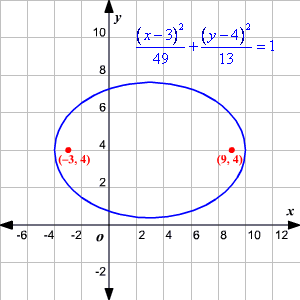An ellipse can also be defined as a conic section obtained by the intersection of a cone with a plane that is not perpendicular to the axis of symmetry and does not intersect the cone’s base.